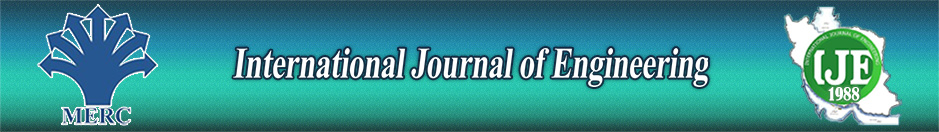Economic Order Quantity for Deteriorating Items with Non Decreasing Demand and Shortages Under Inflation and Time Discounting

Author

Mathematics, Graphic Era University, Dehradun

Abstract

Some products like green vegetables, volatile liquids and others deteriorate continuously due to evaporation, spoilage etc. In this study, an inventory model is developed for deteriorating items with linearly time dependent demand rate under inflation and time discounting over a finite planning horizon. Shortages are allowed and linearly time dependent. Mathematical model is presented for the proposed model. We show that total profit is concave with respect fraction of scheduling period k. The results are discussed with the help of numerical example. A sensitivity analysis of the optimal solution with respect to the key parameters is also discussed.

Keywords